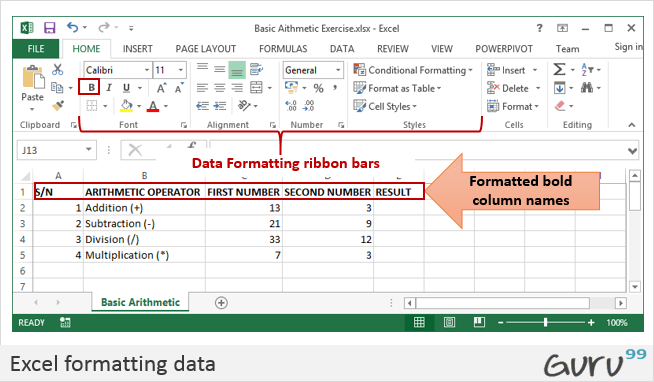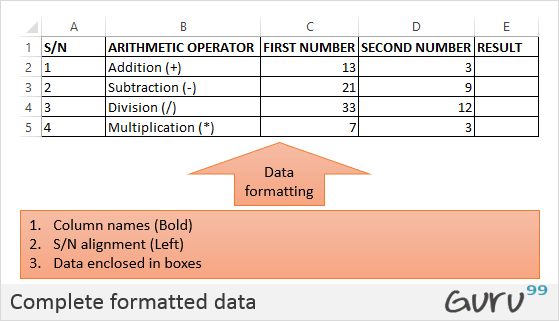In this tutorial, we are going to perform basic arithmetic operations i.e. addition, subtraction, division and multiplication. The following table shows the data that we will work with and the results that we should expect.

 S/N ARITHMETIC OPERATOR FIRST NUMBER SECOND NUMBER RESULT 1 Addition (+) 13 3 16 2 Subtraction (-) 21 9 12 3 Division (/) 33 12 2.75 4 Multiplication (*) 7 3 21

Let's now use Microsoft excel to achieve the above results

Create a folder on your computer in my documents folder and name it Guru99 Excel Tutorials

For this tutorial, we will be using Microsoft Excel 2013. The good news is even if you have Microsoft Excel 2007 or 2010, you will still be able to follow the tutorial and get the same result.

Open Excel. You will get a window similar to the one shown below. The outlook of Excel will depend on your version.

• Enter the data in your worksheet as shown in the image above.
• We will now perform the calculations using the respective arithmetic operators. When performing calculations in Excel, you should always start with the equal (=) sign.
• Let's start with the one for addition. Write the following formula in E2 Excel (Result column)
• =C2+D2

HERE,

• "=" tells Excel to evaluate whatever follows after the equal sign
• "C2" is the cell address of the first number given by C representing the column letter and 2 representing the row number
• "D2" is the cell address of the second number given by D representing the column letter and 2 representing the row number

Press enter key on the keyboard when done. You should get 16 as the result.

### Tutorial exercise 1

Using the knowledge gained in the above example, try to write the formulas for subtraction, division, and multiplication.

## Formatting data in Microsoft Excel

We all love beautiful things don't we? Formatting in Excel helps us achieve exactly that. We can make our spreadsheets more presentable. We will use the data in the arithmetic operations table. We will make the column names;

• Bold
• Align serial numbers to the left
• Enclose the data in boxes.

### Make column names bold

• Highlight the cells that have the column names by dragging them.
• Click on the bold button represented by B command.
• Your workbook should now appear as follows### Align data to the left

• We will align the serial numbers to the left
• Highlight all the data in the S/N column
• Click on align left as shown below

### Enclose data in boxes

Highlight all the columns and rows with data

On the font ribbon bar, click on borders command as shown below.

You will get the following drop down menu

Select the option "All Borders".

Your data should now look as follows### Tutorial Exercise 2

Using the knowledge gained above, try to change the font colour and try out other options available on the Home tab.

## Setting the print area and printing (Print View) & Page Layout

The print area is the part of the worksheet that you would like to print out on paper. The quick and easy way of doing it is by using the following shortcut commands

Ctrl + P

You will get the following print preview.

Press Esc button to exit print preview mode

### Tutorial exercise 3

The page setup ribbon bar has a number of options i.e. orientation, size, etc. Try to apply the different settings and use Ctrl + P shortcut to preview the effects on the worksheet.

## Summary

In this article, we have learnt how to perform basic arithmetic operations using Excel, Formatting data, How to Setting the print area and printing (Print View).

YOU MIGHT LIKE: